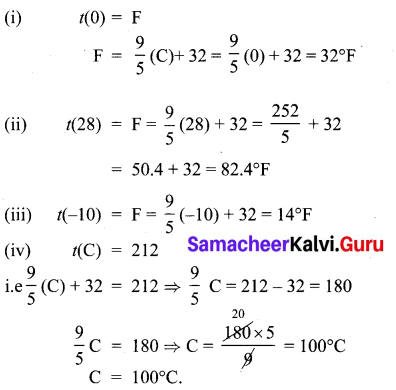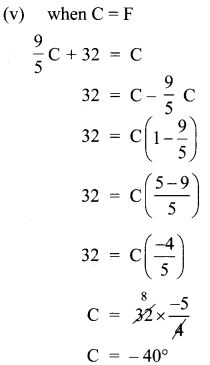## Tamilnadu Samacheer Kalvi 10th Maths Solutions Chapter 1 Relations and Functions Ex 1.4

Exercise 1.4 Class 10 Maths Samacheer Question 1.
Determine whether the graph given below represent functions. Give reason for your answers concerning each graph.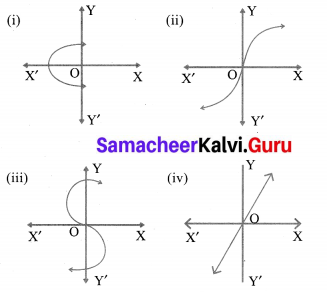Solution: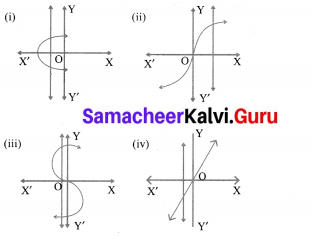(i) It is not a function. The graph meets the vertical line at more than one points.
(ii) It is a function as the curve meets the vertical line at only one point.
(iii) It is not a function as it meets the vertical line at more than one points.
(iv) It is a function as it meets the vertical line at only one point.

10th Maths Exercise 1.4 Samacheer Kalvi Question 2.
Let f :A → B be a function defined by f(x) = $$\frac{x}{2}$$ – 1, Where A = {2, 4, 6, 10, 12},
B = {0, 1, 2, 4, 5, 9}. Represent f by
(i) set of ordered pairs;
(ii) a table;
(iii) an arrow diagram;
(iv) a graph
Solution:
f: A → B
A = {2, 4, 6, 10, 12}, B = {0, 1, 2, 4, 5, 9}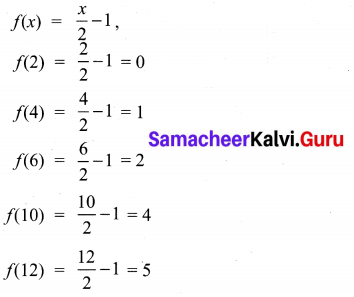(i) Set of ordered pairs
= {(2, 0), (4, 1), (6, 2), (10, 4), (12, 5)}
(ii) a table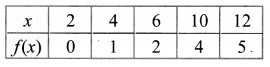(iii) an arrow diagram;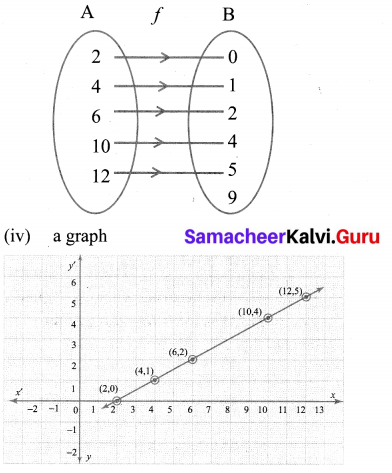Ex 1.4 Class 10 Samacheer Question 3.
Represent the function f = {(1, 2),(2, 2),(3, 2), (4,3), (5,4)} through
(i) an arrow diagram
(ii) a table form
(iii) a graph
Solution:
f = {(1, 2), (2, 2), (3, 2), (4, 3), (5, 4)}
(i) An arrow diagram.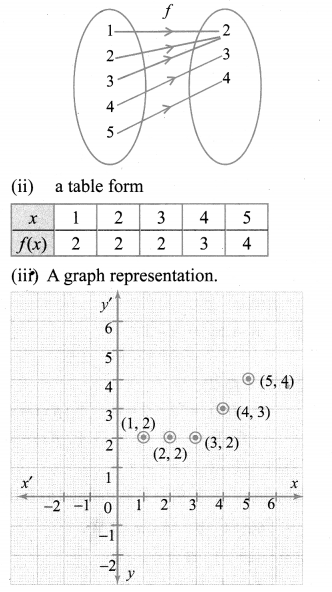10th Maths Exercise 1.4 Question 4.
Show that the function f : N → N defined by f{x) = 2x – 1 is one – one but not onto.
Solution:
f: N → N
f(x) = 2x – 1
N = {1, 2, 3, 4, 5,…}
f(1) = 2(1) – 1 = 1
f(2) = 2(2) – 1 = 3
f(3) = 2(3) – 1 = 5
f(4) = 2(4) – 1 = 7
f(5) = 2(5) – 1 = 9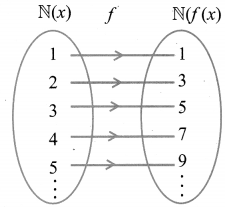In the figure, for different elements in x, there are different images in f(x).
Hence f : N → N is a one-one function.
A function f: N → N is said to be onto function if the range of f is equal to the co-domain of f
Range = {1, 3, 5, 7, 9,…}
Co-domain = {1, 2, 3,..}
But here the range is not equal to co-domain. Therefore it is one-one but not onto function.

10th Maths 1.4 Exercise Question 5.
Show that the function f: N → N defined by f (m) = m2 + m + 3 is one – one function.
Solution:
f: N → N
f(m) = m2 + m + 3
N = {1, 2, 3, 4, 5…..}m ∈ N
f{m) = m2 + m + 3
f(1) = 12 + 1 + 3 = 5
f(2) = 22 + 2 + 3 = 9
f(3) = 32 + 3 + 3 = 15
f(4) = 42 + 4 + 3 = 23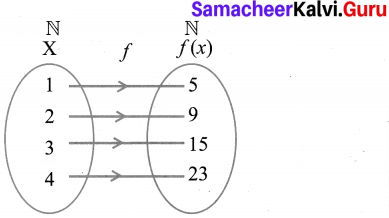In the figure, for different elements in the (X) domain, there are different images in f(x). Hence f: N → N is a one to one but not onto function as the range of f is not equal to co-domain.
Hence it is proved.

10th Maths Ex 1.4 Question 6.
Let A = {1,2, 3, 4} and B = N .
Let f: A → B be defined by f(x) = x3 then,
(i) find the range of f
(ii) identify the type of function
A = {1,2, 3,4}
B = {1,2, 3, 4, 5,….}
f(x) = x3
f(1) = 13 = 1
f(2) = 23 = 8
f(3) = 33 = 27
f(4) = 43 = 64
(i) Range = {1,8, 27, 64}
(ii) one -one and into function.

10th Maths Exercise 1.4 Answers Question 7.
In each of the following cases state whether the function is bijective or not. Justify your answer.
(i) f: R → R defined by f(x) = 2x + 1
(ii) f: R → R defined by f(x) = 3 – 4x2
Solution:
(i) f : R → R
f(x) = 2x + 1
f(1) = 2(1) + 1 = 3
f(2) = 2(2) + 1 = 5
f(-1) = 2(-1) + 1 = -1
f(0) = 2(0) + 1 = 1
It is a bijective function. Distinct elements of A have distinct images in B and every element in B has a pre-image in A.
(ii) f: R → R; f(x) = 3 – 4x2
f(1) = 3 – 4(12) = 3 – 4 = -1
f(2) = 3 – 4(22) = 3 – 16 = -13
f(-1) = 3 – 4(-1)2 = 3 – 4 = -1
It is not bijective function since it is not one-one

Samacheer Kalvi 10th Maths Book Graph Solution Question 8.
Let A = {-1, 1} and B = {0, 2}. If the function f: A → B defined by f(x) = ax + b is an onto function? Find a and b.
Solution:
A= {-1, 1},B = {0, 2}
f: A → B, f(x) = ax + b
f(-1) = a(-1) + b = -a + b
f(1) = a(1) + b = a + b
Since f(x) is onto, f(-1) = 0
⇒ -a + b = 0 …(1)
& f(1) = 2
⇒ a + b = 2 …(2)
-a + b = 0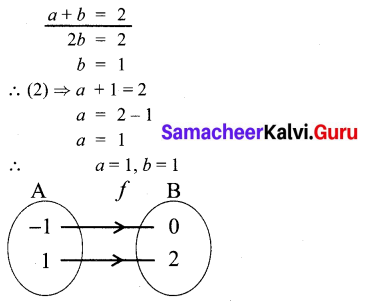10th Maths Exercise 1.4 In Tamil Question 9.
If the function f is defined by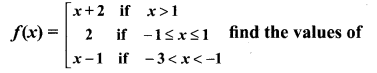(i) f(3)
(ii) f(0)
(iii) f(-1.5)
(iv) f(2) + f(-2)
Solution:
(i) f(3) ⇒ f(x) = x + 2 ⇒ 3 + 2 = 5
(ii) f(0) ⇒ 2
(iii) f (- 1.5) = x – 1
= -1.5 – 1 = -2.5
(iv) f(2) + f(-2)
f(2) = 2 + 2 = 4     [∵ f(x) = x + 2]
f(-2) = -2 – 1 = -3    [∵ f(x) = x – 1]
f(2) + f(-2) = 4 – 3 = 1

Samacheer Kalvi 10th Maths Exercise 1.4 Question 10.
A function f: [-5,9] → R is defined as follows: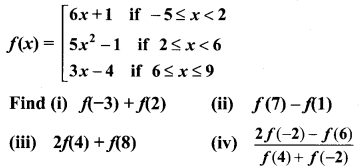Solution:
f : [-5, 9] → R
(i) f(-3) + f(2)
f(-3) = 6x + 1 = 6(-3) + 1 = -17
f(2) = 5 × 2 – 1 = 5(22) – 1 = 19
∴ f(-3) + f(2) = -17 + 19 = 2

(ii) f(7) – f(1)
f(7) = 3x – 4 = 3(7) – 4 = 17
f(1) = 6x + 1 = 6(1) + 1 = 7
f(7) – f(1) = 17 – 7 = 10

(iii) 2f(4) + f(8)
f(4) = 5x2 – 1 = 5 × 42 – 1 = 79
f(8) = 3x – 4 = 3 × 8 – 4 = 20
∴ 2f(4) + f(8) = 2 × 79 + 20 = 178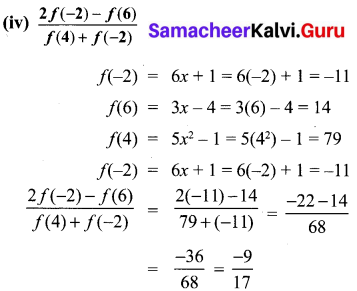10th Maths 1.4 Question 11
The distance S an object travels under the influence of gravity in time t seconds is 1 2 given by S(t) = $$\frac { 1 }{ 3 }$$gt2 + at + b, where, (g is the acceleration due to gravity), a, b are constants. Check if the function S(t) is one-one.
S(t) = $$\frac { 1 }{ 2 }$$gt2 + at + b
Let the time be 1, 2, 3 …. n seconds
S(1) = $$\frac { 1 }{ 2 }$$g(1)2 + a(1) + b
= $$\frac { g }{ 2 }$$ + a + b
S(2) = $$\frac { 1 }{ 2 }$$ g(2)2 + a(2) + b
= $$\frac { 4g }{ 2 }$$ + 2a + b
= 2g + 2a + b
S(3) = $$\frac { 1 }{ 2 }$$ g(3)2 + a(3) + 6
= $$\frac { 9 }{ 2 }$$ g + 3a + b
For every different value of t, there will be different distance.
∴ It is a one-one function.

Samacheer Kalvi 10th Maths Book Graph Solutions Question 12.
The function ‘t’ which maps temperature in Celsius (C) into temperature in Fahrenheit (F) is defined by t(C)= F where F = $$\frac{9}{5}$$ C + 32 . Find,
(i) t(0)
(ii) t(28)
(iii) t(-10)
(iv) the value of C when t(C) = 212
(v) the temperature when the Celsius value is equal to the Farenheit value.
Solution: